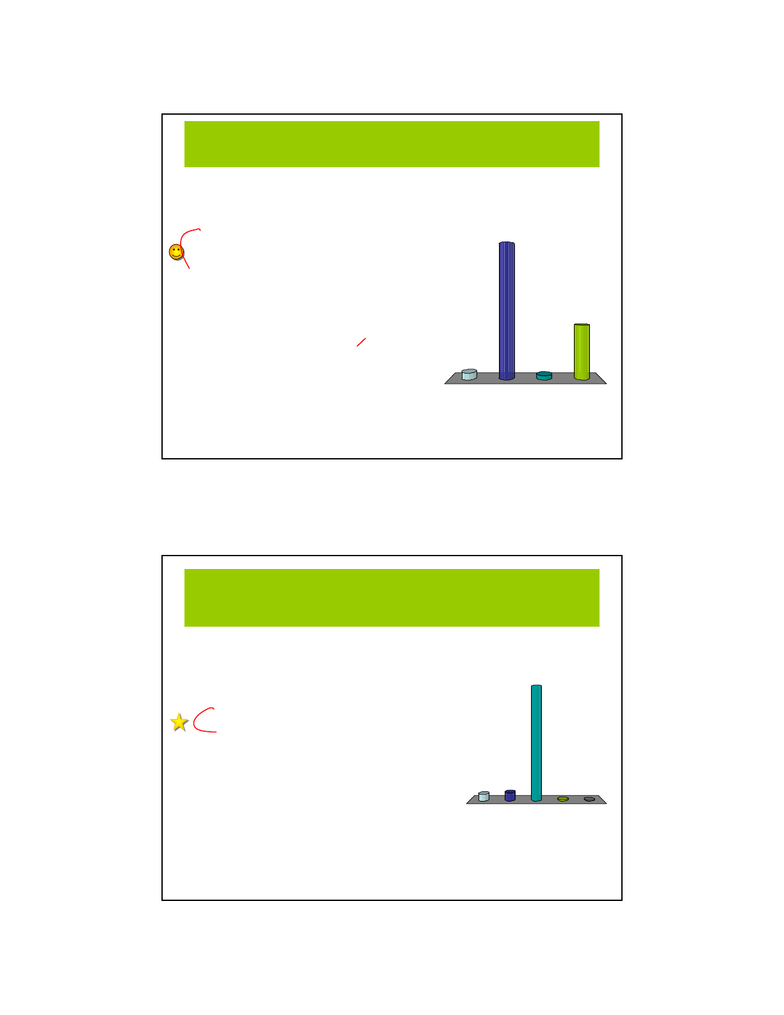Study Guides (390,000)
CA (150,000)
McGill (6,000)
Physics (100)
Midterm

# PHYS 131 Midterm Review_Summary (Chapter1-8).pdf

Department
Physics
Course Code
PHYS 131
Professor
Kenneth Ragan
Study Guide
Midterm

This preview shows pages 1-3. to view the full 12 pages of the document.1
PHYS 131 - Review: Ch. 1 to 8 1
Midterm review
First, choose a team…
The Newtons
The Joules
The Watts
The Galileans
The Hookes
44
25
55
67
43
1. The Newtons
2. The Joules
3. The Watts
4. The Galileans
5. The Hookes
PHYS 131 - Review: Ch. 1 to 8 2
Chapter 1: Preliminaries
• units
• significant figures, order of magnitude estimates
• dimensional analysis / sanity checks / diagrams
Chapter 2: 1-D Kinematics
• speed vs velocity / distance vs displacement
• average (speed/acceleration) vs instantaneous
• graphical approach to disp., vel., and acceleration.
• special case of motion at constant acceleration (eg. freefall):
v = v0+ at
vaver = (v + v0)/2
v2= v02+ 2a (x – x0)
x = x0+ v0t + ½at2
PHYS 131 Review: Chapters 1 – 8

Only pages 1-3 are available for preview. Some parts have been intentionally blurred.2
PHYS 131 - Review: Ch. 1 to 8 3
An acceleration vector:
Tells you how ...
Has a directio...
Points in the ...
Is parallel or...
8
64
5
161
1. Tells you how fast an
object is moving
2. Has a direction that can be
determined from two
velocity vectors
3. Points in the direction of
motion
4. Is parallel or opposite to
the direction of motion
PHYS 131 - Review: Ch. 1 to 8 4
In 1-D motion, the slope at a point on a position-vs-time
graph of an object is
The objects speed at...
The object’s average ...
The object’s instanta...
The objects accelera...
The distance travelle...
12 15 12
215
1. The object’s speed at that
moment
2. The object’s average velocity
at that moment
3. The object’s instantaneous
velocity at that moment
4. The object’s acceleration at
that moment
5. The distance travelled by
the object to that point

Only pages 1-3 are available for preview. Some parts have been intentionally blurred.3
PHYS 131 - Review: Ch. 1 to 8 5
Chapter 3: Vectors
• vectors vs scalars
• vector components; unit vectors
Chapter 4: Motion in two dimensions
• Position and velocity vectors
• Derivatives by components
• 2-D motion at constant acceleration
• Projectile motion:
• parabolic shape
• range equation
• Uniform circular motion
• Relative velocities (frames of reference)
v = v0+ at
vaver = (v + v0)/2
v2= v02+ 2a (x – x0)
x = x0+ v0t + ½at2
dproj = 2 (vy0vx0)/g
dproj = v2sin(2Θ)/g
a = v2/r
PHYS 131 - Review: Ch. 1 to 8 6
Which of the following is true?
A component of a vect..
A component of a vect..
A component of a vec...
8
47
191
1. A component of a vector is
always larger than the
magnitude of the vector.
2. A component of a vector is
never larger than the
magnitude of the vector.
3. A component of a vector is
sometimes larger than, and
sometimes smaller than, the
magnitude of the vector.